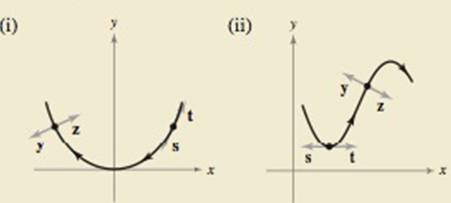Chapter 12.4, Problem 48E

Chapter
Section
Textbook Problem
1 views

# HOW DO YOU SEE IT? The figures show the paths of two policies.(a) Which vector. s or t. represents the unit tangent vector? Explain.(b) Which vector, y or z represents the principal unit normal vector? Explain.

(a)

To determine
Whether vector s or t represents the unit tangent vector.

Explanation

Given:

Explanation: The above graphs in the above gr...

(b)

To determine
Whether vector y or z represents the principal unit normal vector.

### Still sussing out bartleby?

Check out a sample textbook solution.

See a sample solution

#### The Solution to Your Study Problems

Bartleby provides explanations to thousands of textbook problems written by our experts, many with advanced degrees!

Get Started